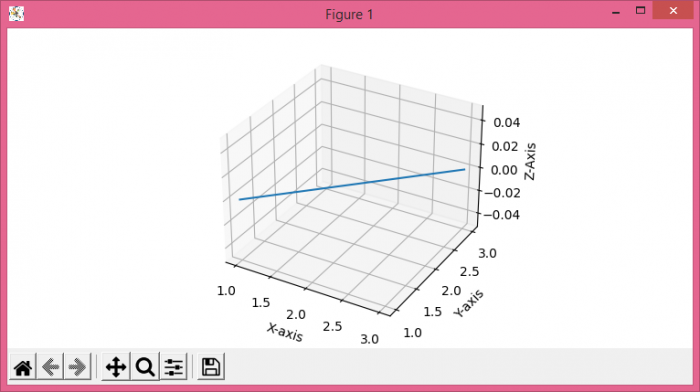# Tweaking axis labels and names orientation for 3D plots in Matplotlib

To tweak axis labels and names orientation for 3D plots in matplotlib, we can take the following steps −

• Set the figure size and adjust the padding between and around the subplots.
• Create a new figure or activate an existing figure with facecolor=white.
• Get the current figure with 3d projection.
• Set X, Y and Z Axis labels with linespacing.
• Plot the data points using plot() method.
• Set the axis distance.
• To display the figure, use show() method.

## Example

import matplotlib.pyplot as plt

plt.rcParams["figure.figsize"] = [7.50, 3.50]
plt.rcParams["figure.autolayout"] = True

figure = plt.figure(facecolor='w')
ax = figure.gca(projection='3d')

xLabel = ax.set_xlabel('X-axis', linespacing=3.2)
yLabel = ax.set_ylabel('Y-axis', linespacing=3.1)
zLabel = ax.set_zlabel('Z-Axis', linespacing=3.4)

plot = ax.plot([1, 2, 3], [1, 2, 3])
ax.dist = 10

plt.show()

## Output